# 9 “new math” problems and methods## ByThe Understood Team

Are you confused by unfamiliar-looking math problems in your child’s homework? The approach to teaching math has changed in recent years. The examples below, created with the help of math specialist Heidi Cohen, can help you help your child with “new math.”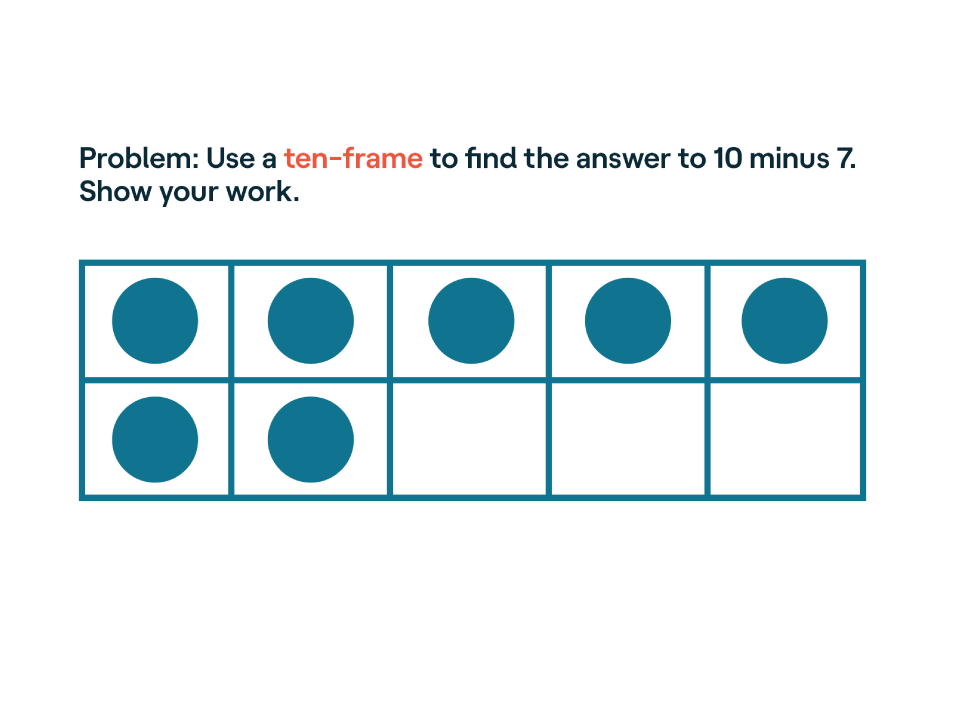## Ten-frame

A ten-frame is a set of 10 boxes with dots in some or all of the boxes. Kids can see how different combinations of numbers add up to 10. The ten-frame is especially good for showing how subtraction works.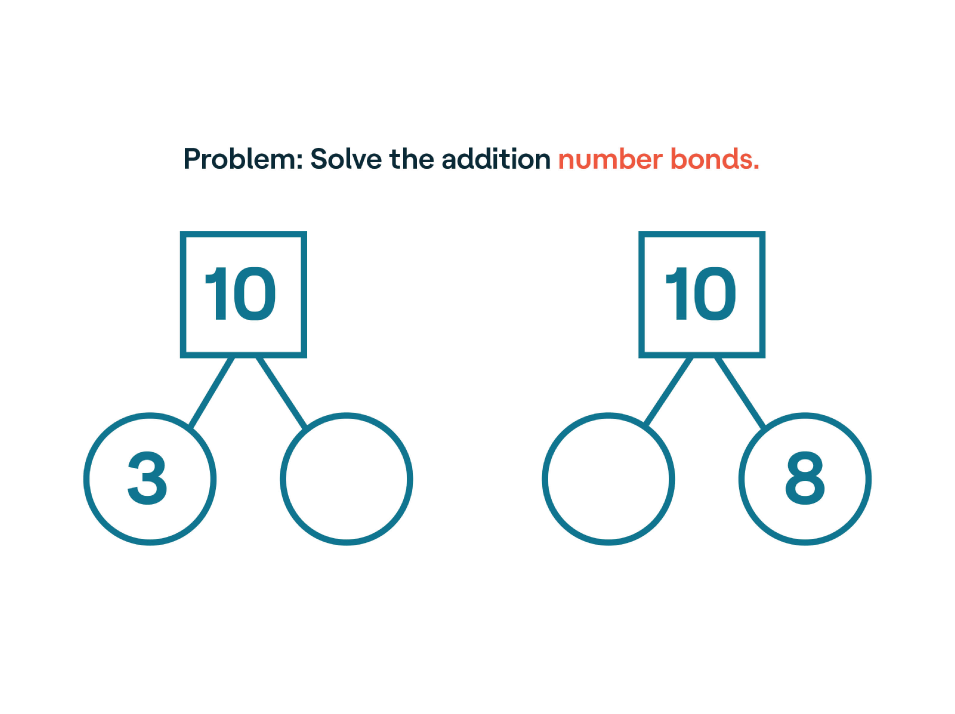## Number bond

A number bond uses lines to link a group of numbers together, showing how they are related. In the first drawing, the relationship between the numbers 3 and 10 is shown by adding the number 7 to the empty circle (3 + 7 = 10). This helps kids see how a single number can be broken down into smaller parts.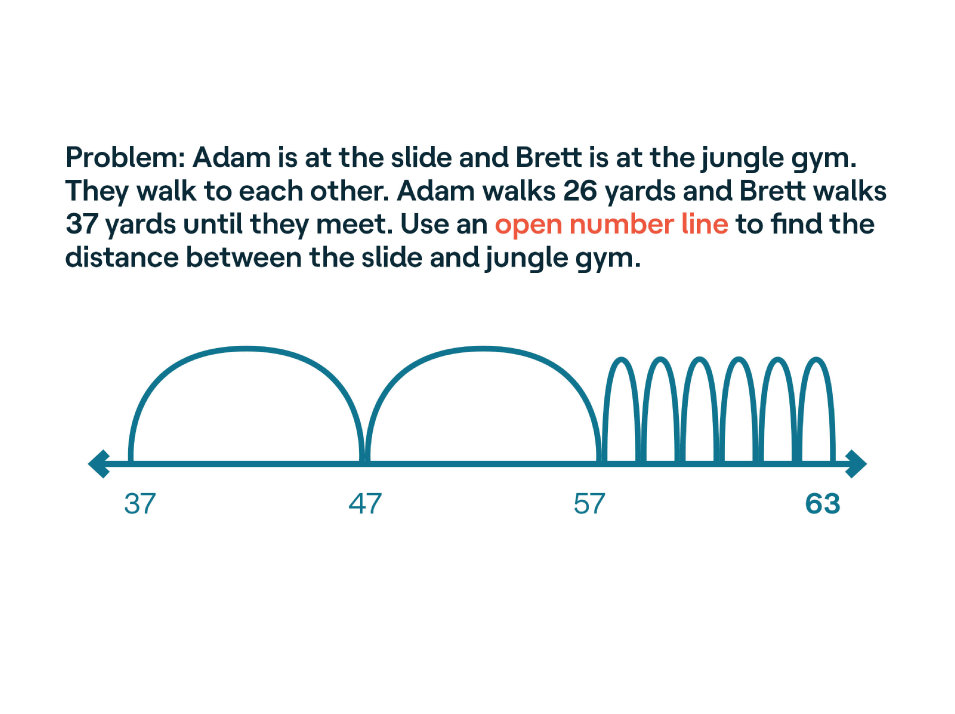## Open number line

An open number line has no numbers already written in. The student can use any number as the starting place. (Here, 37 is the starting place because that is how many yards Brett walked. The 26 yards that Adam walked are then added.) The open number line lets kids add or subtract in a visual way. It is often used to help solve word problems.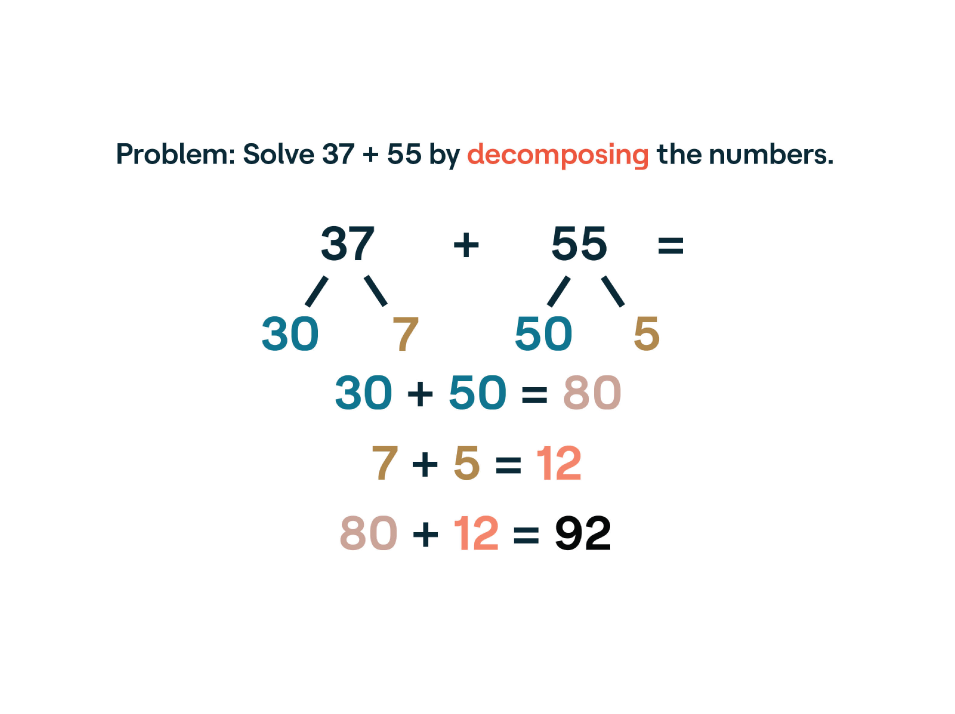## Decomposing (also called “expanded form”)

Decomposing is a strategy to solve math problems by breaking a number down into its digit values. For example, 37 becomes 30 and 7. Once you break the number down, you can add or subtract the individual digit values to get the answer.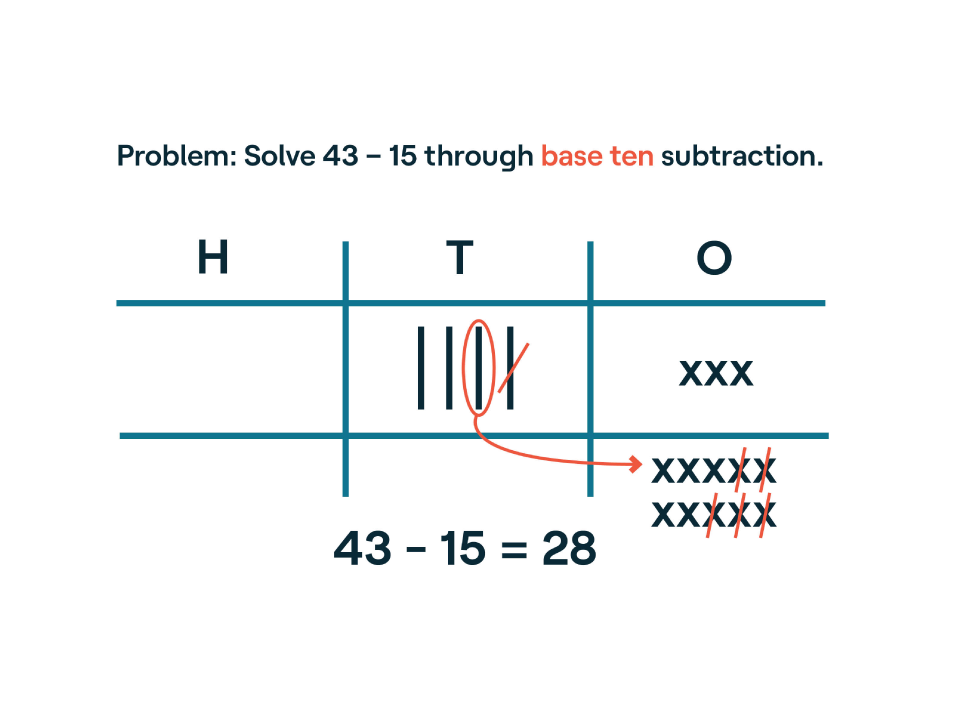## Base ten

Base ten is a strategy to solve addition and subtraction problems by using a table divided into hundreds, tens, and ones. You’ll probably see the term “regrouping” used for this method. Each number goes into the chart according to its place value. For example, 43 would mean 4 tens and 3 ones. This helps kids see when to “borrow” and “carry” numbers from one place value to another.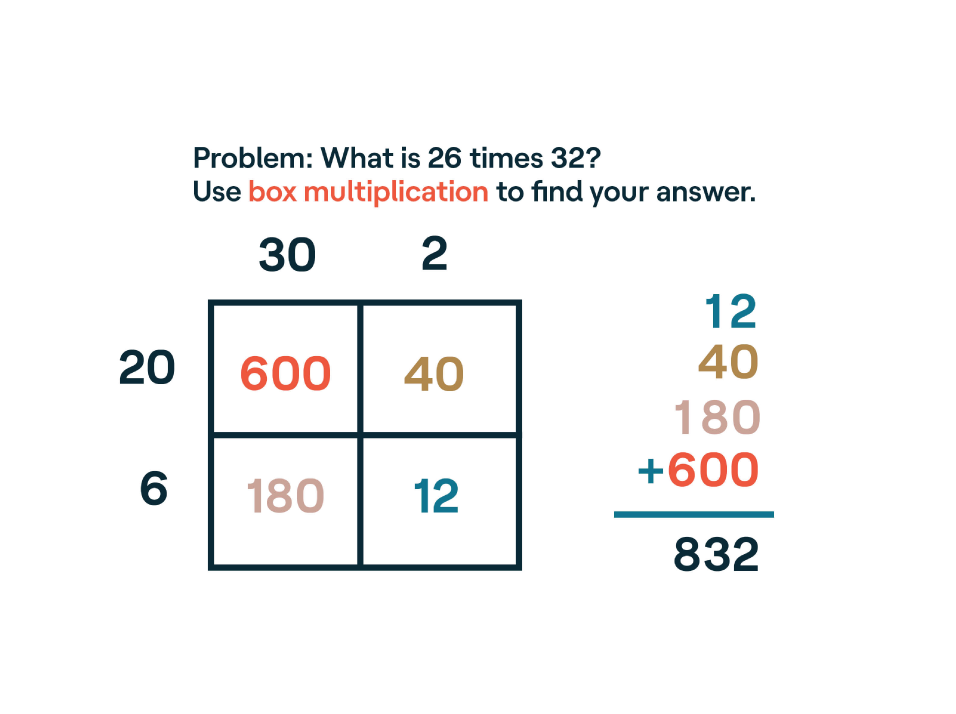## Box multiplication

Box multiplication is a method of breaking numbers down into digit values. In a table, the numbers are broken down by value and multiplied separately. After each number has been multiplied, the total values are added together. This method can be helpful for kids who have trouble with traditional multiplication using larger numbers.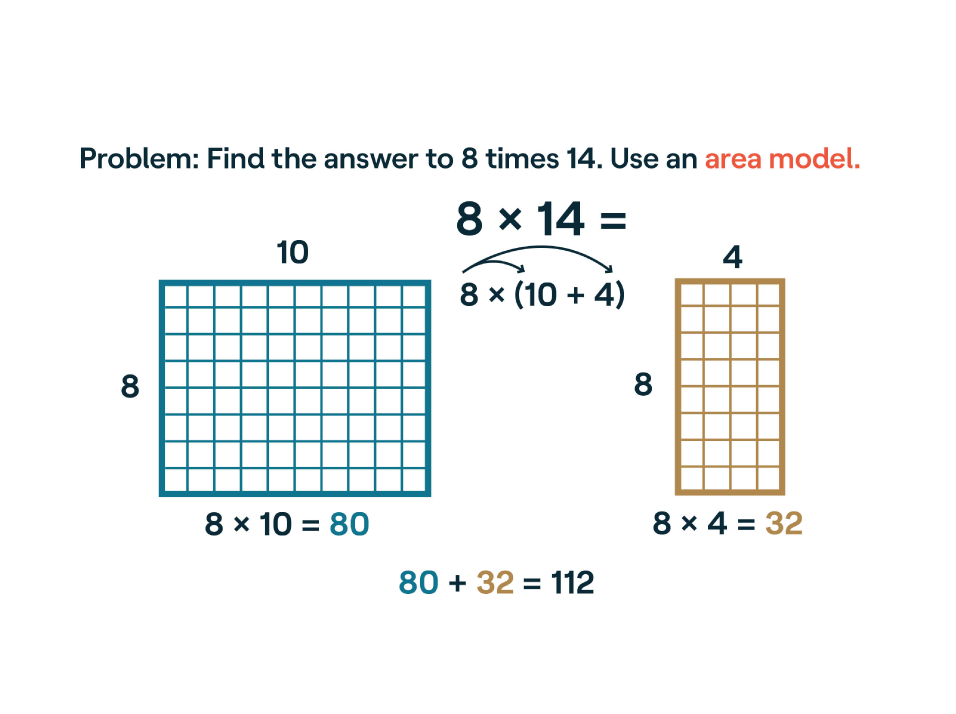## Area model

An area model uses the length and width of a rectangle or square to break down a multiplication problem. Each shape is calculated and the answers are added together. It’s another way to make math more visual for kids.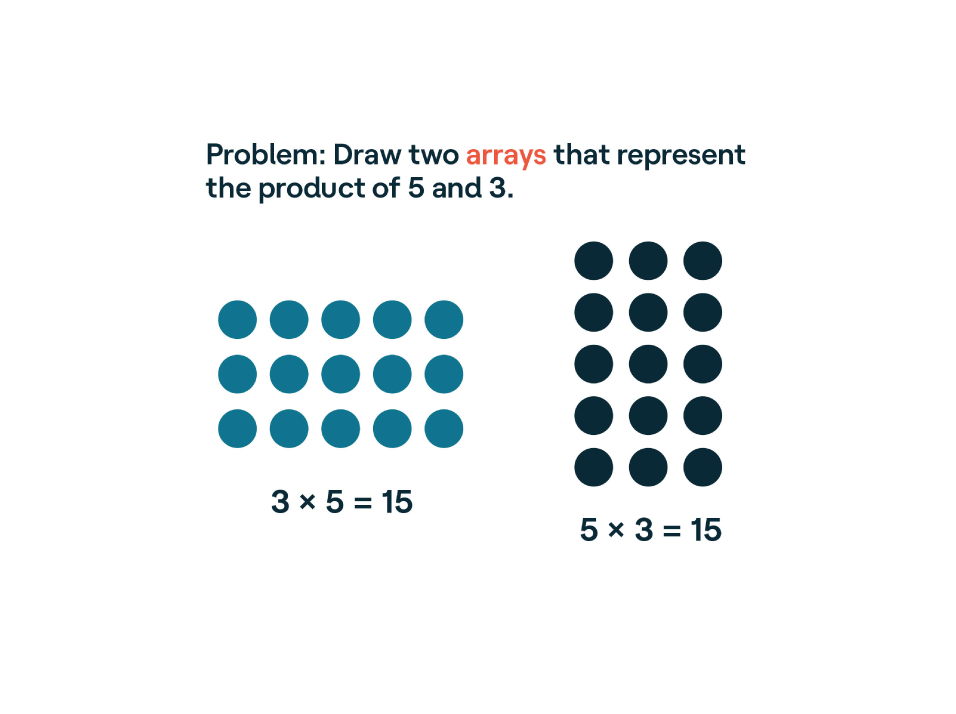## Array

Like an area model, an array is an arrangement of objects that represent a number. This model is often used to help kids see the different qualities of addition and multiplication.## Bar modeling (also known as a “tape diagram”)

A bar model uses bars to visually represent numbers and unknowns in a word problem. It can help kids see how quantities compare to each other. Kids can adapt a bar model to solve many kinds of problems.

## Share